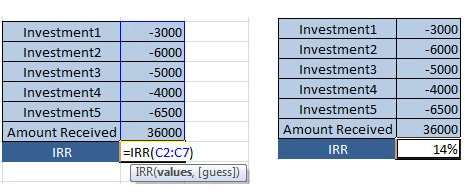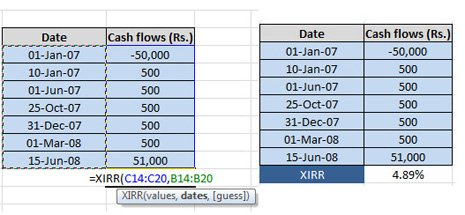HomeInvestmentCalculating Returns of Investment - Formulas & Examples - 2

# Calculating Returns of Investment – Formulas & Examples – 2We have discussed about absolute returns, simple annualized returns & compounded annual growth rate in pervious article Calculating Returns of Investment – Formulas & Examples – 1. Return calculations looked so fare in previous article was done using value of investment at beginning and at the end of period. But it does not tell actual story in terms of comparison with other investment option. So we are herewith article explaining Rolling returns, relative returns, peer returns, IRR and XIRR.

#### Rolling Returns:-

Suppose someone has purchased two mutual funds, A and B, both purchased with NAV of 12 and both sold seven years later at NAV of 32 as shown in figure below.Absolute Returns and CAGR in this case is same

• Point-to-point returns: Both have generated an absolute return of 100* (32-12)/32 =166.67%.
• CAGR :CAGR calculation would also yield identical results, that is, 10.41%

Although CAGR is same you can see their respective performance-A is consistently rising whereas B, after showing a robust growth for the first four years, declines consistently after 2009. Clearly, A is a better choice, which you’d never know by relying on the point-to-point/CAGR return measures.

Rolling returns is nothing but dividing time frames in equal but small part and taking average of small period returns. This gives information about performance of asset class over time period.

We have come across HDFCFund: Sensex rolling return calculator this calculator can calculate rolling returns of Sensex from any date between 12 Nov 1997 to 12 Nov 2012.

But rolling period statistics have their limitations, too. By their very nature, they tend to put more weight on returns in the middle of the measurement period and less on those at either end.

#### Relative Return:-

Relative returns enable us to know the true return earned by the fund over and above the benchmark. It determines how the return of a given stock or fund compares to that of benchmark. This can be useful in making investment decisions. For example, if the stock you are holding achieves a return of 20 per cent, while the benchmark index say Nifty managed 15.58 per cent, then the stock has achieved a relative return of a +4.42 per cent. A stock that falls less than the benchmark in a falling market is considered to have done well, as it manages to contain losses for the investor.

#### Peer Returns:-

Suppose you invest in State Bank of India (SBI) and the stock increases in a year by 7%. Other banking stocks rise like HDFC Bank by 9% ICICI Bank by 6.5%, Punjab National Bank (PNB) by 5% and Banking Index by 6%. It means SBI has given better returns as compared to Banking Index and among its peers better than PNB but less than HDFC Bank and ICICI bank. Peer return helps in selection of investment within a particular sector or sub-group of an asset class such as banking stock in above example.

So far we focused on investing only one time. But How do you calculate your returns when you invest some amount every year/or at some time period and at the end you receive your money back or in between you get some dividend? That’s where you required IRR & XIRR.

#### Internal rate of Return(IRR):-

Suppose your invest 3,000 , 6,000 , 5,000 , 4,000 and 6,500 in 5 yrs and Get 36,000 at the end of 5 yrs , what is your Return ? Or you invested Rs 10,000 in the stock which gave 10% annual dividends. At end of 5 years you sell stock for 12,000 Rs. The return that is used in such cases is IRR or Internal Rate of Return.

IRR is Internal Rate of Return and it is used to calculate the returns given some amount at a fixed interval i.e. after every 3 months or after every 1 yr. The only thing which matters is that there should be equal distance between two installments.

#### IRR Calculation:-

Let’s try to find IRR in an Excel sheet, first enter the original amount invested. The amount invested should be represented by a ‘minus’ sign. In each cell enter the cash flows which received each year. Remember to include the ‘minus’ sign whenever you invest money. Now find out IRR by mentioning =IRR(values,[guess])IRR for investment in this case is equal to 14% as shown in picture above.

#### External Internal Rate of Return (XIRR):-

If you make an investment in the beginning of the investment period and you receive cash flows that are ‘not’ necessarily periodic such as dividends offered on mutual fund units. In this case how would you calculate the return on your investment?  You can calculate returns by means of using powerful function XIRR of Excel.

#### XIRR Calculation:-

Suppose you invest 50,000 Rs in mutual funds and you get 500 Rs dividend at different interval and you sold these mutual funds at value of 51,000 Rs. Let’s try to find XIRR of this example using Excel.

In an Excel sheet, first enter the original amount invested. The amount invested should be represented by a ‘minus’ sign. In each cell enter the cash flows which received each period. Remember to include the ‘minus’ sign whenever you invest money. Now find out XIRR by mentioning =XIRR(values, dates,[guess])XIRR for investment in this case is equal to 4.89% as shown in picture above.

I hope I have done my job by explaining various ways to calculate returns. So next time before doing any investments please remember what you read here and apply your wisdom to calculate returns. Be smart investor, & take investment decision based on calculation and not assumptions.

Comments ? I would love to hear if these concepts mention here are of any use to you.